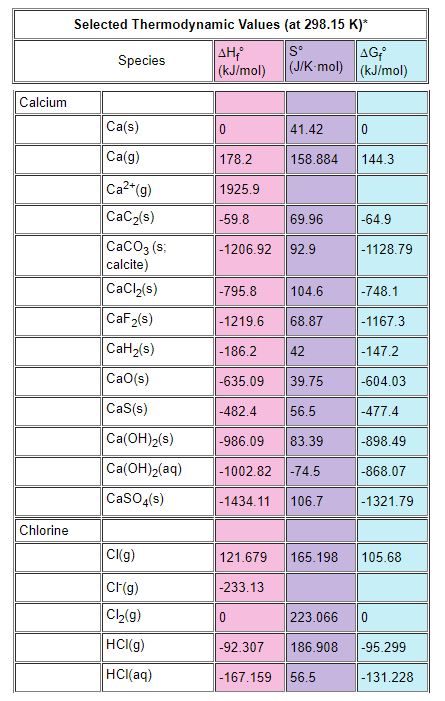# Problem: Use the table below to answer the questions that follow.The value of ΔG° at 25 °C for the formation of calcium chloride from its constituent elements, Ca(s) + Cl2(g) --&gt; CaCl2(s)a. 0 kJb. -741.8 kJc. +741.8 kJd. -892.4 kJe. 369.1 kJ

###### FREE Expert Solution

We’re being asked to determine the ΔG for the given reaction:

Ca(s) + Cl2(g) → CaCl2(s)

We can use the following equation to solve for ΔG˚rxn:

80% (233 ratings)###### Problem Details

Use the table below to answer the questions that follow.The value of ΔG° at 25 °C for the formation of calcium chloride from its constituent elements, Ca(s) + Cl2(g) --> CaCl2(s)

a. 0 kJ

b. -741.8 kJ

c. +741.8 kJ

d. -892.4 kJ

e. 369.1 kJ